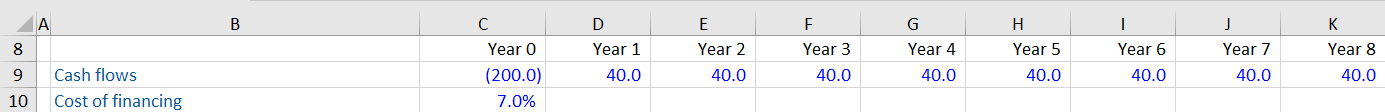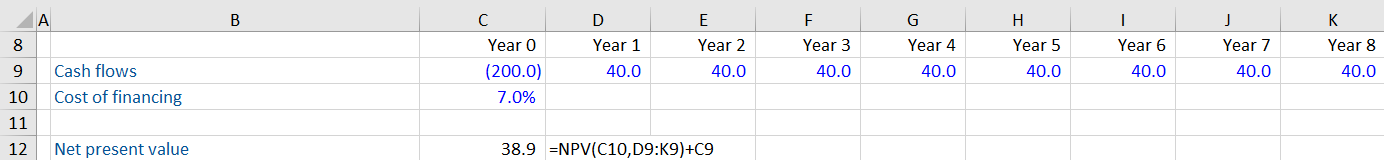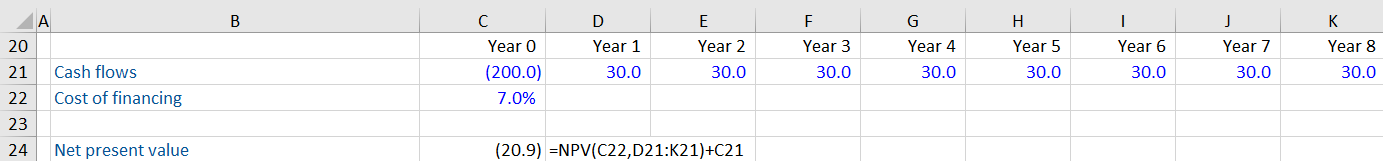## What is Net Present Value?

Net present value (NPV) is one of the most important concepts in valuation & capital budgeting. It is used to evaluate investment opportunities on the present value of future cash outflows and inflows.

## Key Learning Points

• Net present value is calculated as the sum of a discounted series of future cash flows less the cost of the initial investment
• Excel has a function to quickly calculate the net present value =NPV
• It is a financial concept used in valuation and capital budgeting assignments
• A positive NPV is favorable as it indicates the project is profitable i.e. the sum of the future cash flows discounted based on a predefined discount rate is higher than the cost of the initial investment
• It is important to remember than NPV is reliant on the accuracy of the forecasted cash flows being analyzed; these may be difficult to predict accurately which skew the investment decision

## NPV Formula

NPV can be calculated in Excel using a function that hides most of the calculation away. To better understand what is happening in the background, here is an explanation of the formula:

NPV = Present value of expected cash inflows – Present value of cash outflows

If the result is positive, it indicates the project will be profitable. A negative NPV indicates the project is likely to be loss-making, i.e. the present value of cash outflows is more than the cash inflows.

## Calculating NPV in Excel Example

Let us assume a company is planning to buy a plant for 200. The plant is expected to generate revenues of 40 every year for the next 8 years. The discount rate or the cost of financing for this project is 7%. What is the NPV be for this project?

The first step is to determine the number of periods. You can do this by populating rows in Excel with the number of years for this investment. Each year populates a different column. In the above example, Year 0 is when the investment or cash outflow occurs. And in years 1 to 8, the business receives cash inflows. This is a simple example. In the real world, the subsequent years can see both cash inflows and outflows.Next, you use the NPV function in Excel as:

NPV (Cost of financing, Cash flows in Year 1:Year8) + Cash flow in Year 0

Notice you are not including year 0 in the NPV formula. That is because the investment is happening today, and so the present value of the cash outflow is 200 and is already known. There is no need to apply a discount factor to get the present value of this investment. Because all the other inflows are occurring in the future, you need to apply the NPV formula.

The result is a positive number, indicating net cash inflows of 38.9. This is a profitable investment and is worth considering.Suppose the investment provides a lower return of 30 each year. What is the Net Present Value for this project?Using the previous formula, you get a negative net present value of -20.9. This indicates the project is likely to lose cash over time and should not be considered.

## Key Limitation

The NPV method is dependent entirely on estimates of future cash flows. In reality, it is not easy to estimate future cash flows with certainty. Different factors such as market demand, competition, changes in technology, etc could prove the initial assumptions wrong. To make the most out of NPV, care should be taken to create multiple scenarios of future cash flows. Estimates of future cash flows should factor in both the best-case and worst-case scenarios.

## Difference Between Present Value and Net Present Value

Both present value and net present value are used for estimating today’s value of future cash flows. A key difference is that the NPV function in Excel can factor in cash flows that change over time, whereas the PV function assumes static cash flows that occur at evenly spaced intervals.